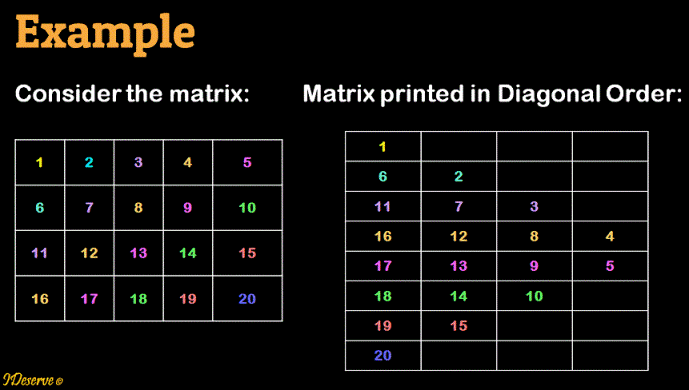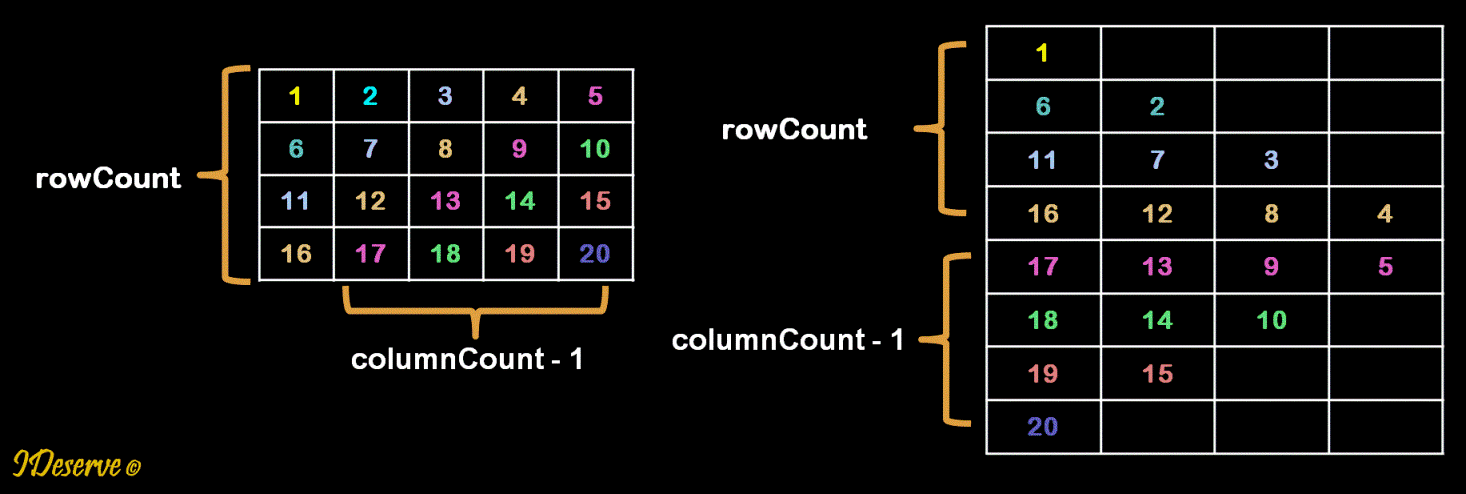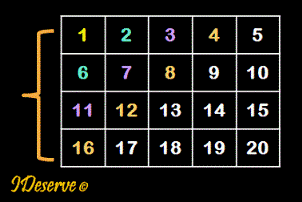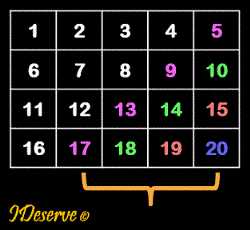# Print a Matrix Diagonally

Given a matrix of mxn dimensions, print the elements of the matrix in diagonal order.Please check out the video below for detailed explanation of the algorithm with animations.

## Algorithm/Insights

rowCount = number of rows
columnCount = number of columns
Then, number of diagonals will be = rowCount + columnCount - 1 as depicted in the diagram below.Step 1: Print first rowCount diagonals
Print diagonals that start from the first column
elements.Step 2: Print next columnCount - 1 diagonals
Print diagonals that start from the last row
elements.Step 1 details: Print first rowCount diagonals
Iterate to print diagonals from row k = 0 to rowCount - 1.
2: Print the element matrix[row][col]
3: Decrement row by 1, increment col by 1 till row >= 0 and  col < columnCount

Step 2 details: Print next columnCount - 1 diagonals
Iterate to print diagonals from column k = 1 to columnCount - 1
1: Start with last row, row = rowCount - 1 and col = k
2: Print the element matrix[row][col]
3: Decrement row by 1, increment col by 1 till row >= 0 and  col < columnCount

## Code Snippet

```
package com.ideserve.questions.saurabh;

import java.util.Arrays;

/**
* <b>IDeserve <br>
* Given a matrix of mxn dimensions, print the elements of the matrix
* in diagonal order.
*
* @author Saurabh
*/
public class PrintMatrixDiagonally {

public static void printMatrixDiagonally(int[][] matrix) {

int row, col;
int rowCount = matrix.length;
int columnCount = matrix.length;

for (int k = 0; k < rowCount; k++) {
for (row = k, col = 0; row >= 0 && col < columnCount; row--, col++) {
System.out.print(matrix[row][col] + " ");
}
System.out.println();
}

for (int k = 1; k < columnCount; k++) {
for (row = rowCount - 1, col = k; row >= 0 && col < columnCount; row--, col++) {
System.out.print(matrix[row][col] + " ");
}
System.out.println();
}
}

public static void main(String[] args) {
int row = 4, col = 5;
int k = 1;
int matrix[][] = new int[row][col];
for (int i = 0; i < row; i++) {
for (int j = 0; j < col; j++) {
matrix[i][j] = k++;
}
}

System.out.println("Input Matrix");
for (int i = 0; i < matrix.length; i++) {
System.out.println(Arrays.toString(matrix[i]));
}

System.out.println("\nPrinting Matrix Diagonally");
printMatrixDiagonally(matrix);
}

}
```

## Order of the Algorithm

Time Complexity is O(mn)
Space Complexity is O(1)